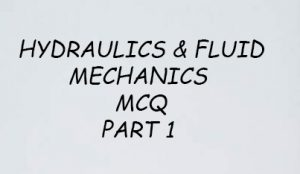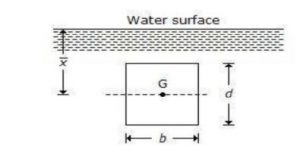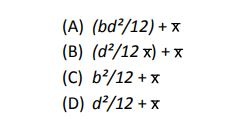# FLUID MECHANICS AND HYDRAULICS MCQ PART 1- IES, RRB, GATE AND PSC

## FLUID MECHANICS AND HYDRAULICS MCQ PART 1 QUESTIONSQuestion No. 01
When a body is placed over a liquid, it will sink down if
(A) Gravitational force is equal to the up-thrust of the liquid
(B) Gravitational force is less than the up-thrust of the liquid
(C) Gravitational force is more than the up-thrust of the liquid
(D) None of the above

Question No. 02
The property by virtue of which a liquid opposes relative motion between its different layers is called
(A) Surface tension
(B) Coefficient of viscosity
(C) Viscosity
(D) Osmosis

Question No. 03
A weir is said to be narrow-crested weir, if the width of the crest of the weir is __________ half the height of water above the weir crest.
(A) Equal to
(B) Less than
(C) More than
(D) None of these

Question No. 04
Euler’s dimensionless number relates the following
(A) Inertial force and gravity
(B) Viscous force and inertial force
(C) Viscous force and buoyancy force
(D) Pressure force and inertial force

Question No. 05
When a body floating in a liquid, is displaced slightly, it oscillates about
(A) C.G. of body
(B) Center of pressure
(C) Center of buoyancy
(D) Metacentre

Question No. 06
The pressure of the liquid flowing through the divergent portion of a Venturimeter
(A) Remains constant
(B) Increases
(C) Decreases
(D) Depends upon mass of liquid

Question No. 07
When the Mach number is between __________ the flow is called super-sonic flow.
(A) 1 and 2.5
(B) 2.5 and 4
(C) 4 and 6
(D) 1 and 6

Question No. 08
In an immersed body, centre of pressure is
(A) At the centre of gravity
(B) Above the centre of gravity
(C) Below be centre of gravity
(D) Could be above or below e.g. depending on density of body and liquid

Question No. 09
A vertically immersed surface is shown in the below figure. The distance of its centre of pressure
from the water surface isQuestion No 1: C

Question No 2: C

Question No 3: B

Question No 4: D

Question No 5: D

Question No 6: C

Question No 7: D

Question No 8: C

Question No 9: B

Open